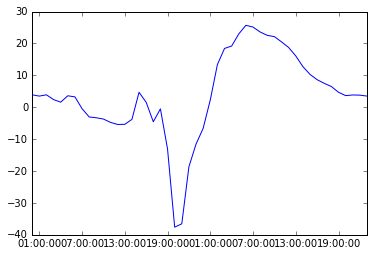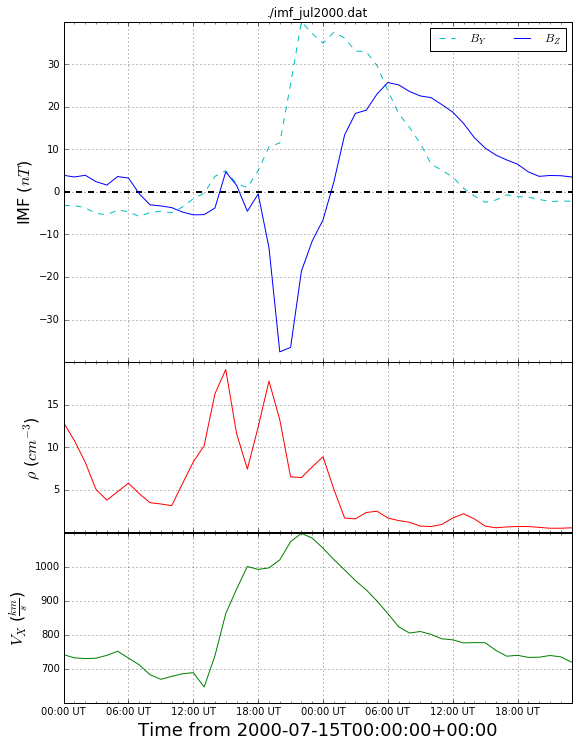An Illustrative Python Example¶

Let's quickly see how we can use Python software to investigate a simple ascii data file.

For this demonstration, we will be interacting with Python using the IPython terminal. This is an enhanced terminal with far more capabilities than the default Python terminal interface. You can use IPython by starting it from the shell terminal, via a Jupyter notebook, or through several Python IDEs. This example is documented via a Jupyter notebook.

Here's our situation: we have ASCII formated solar wind files that are input for the Space Weather Modeling Framework. We want to visualize and manipulate this data. In this brief example, we'll first do this in interarctively via the IPython interface, then we'll do this in a script-like fashion.

Here's what the files look like:

In :
more imf_test.dat

A quick aside: because we're using IPython, we can use simple shell commands, such as ls, more, cp, rm, and others. There's much more you can do with IPython, including interactive debugging and help, command history, and tab-complete. But back to the point at hand...

We want to be able to organize this data into Numpy arrays so that we can perform operations and visualize it using Matplotlib. Fortunately, most of the work is already done for us via a python module called example_imf.py. If you have not already, download this file and place it into the same directory as this notebook file.

Let's start by importing the module. This makes the contents of this file available to us.

In :
import example_imf  # Note that the '.py' is dropped.

A module is just a Python source code file. When we import it, Python executes every line of code within that file. To save time, if we import the file again, Python will not re-execute it. It is possible to reload modules to refresh it after the source code contents have changed.

Using IPython, it is very easy to explore the contents of this module without reading the source code. Let's see if there is any documentation...

In :
example_imf?

The quesion mark syntax is IPython specific, but similar behavior can be achieved via help(example_imf). The text that is displayed is the docstring for the module -- the block of text preceeding any other commands or definitions.

If we want to see what is inside this module, we use module-dot- syntax. For example, let's look at the docstring for a defined class:

In :
example_imf.ImfData?

The dot syntax indicates that ImfData is inside the namespace of the example_imf module. A variable named ImfData is different than exammple_imf.ImfData. It is possible to import specific items out of a certain module or namespace into the global namespace (from example_imf import ImfData). It is also possible to dump the contents of a module into the current namespace (from example_imf import *). DO NOT DO THIS. This is a very poor programming practice.

We now see that there is a class that can read and manipulate our solar wind data. Let's instantiate a new object of class example_imf.ImfData:

In :
data = example_imf.ImfData('./imf_test.dat')  # We can tab-complete the file name, too!
print(data)
ImfData object of ./imf_test.dat

The docstring tells us that this object works like a python dictionary object. In short, this means that we can access values within our dictionary-like object via keys, which are the names of the variables.

In :
print(data.keys()) # ...where keys is an object method of data.
['temp', 'rho', 'time', 'vx', 'vy', 'vz', 'bx', 'by', 'bz']
In :
print( data['rho'] ) # View the entire Numpy array corresponding to 'rho'
[  5.  15.   7.  10.]
In :
print( data['rho'].max() ) # View the max value of data['rho']
15.0

We can also see the times associated with each data entry. Python has a powerful class for handling dates and times, appropriately named datetime objects. These can be stored within a Numpy array.

In :
print( data['time'] )
[datetime.datetime(1998, 1, 1, 1, 0) datetime.datetime(1998, 1, 1, 5, 0)
datetime.datetime(1998, 1, 1, 12, 0) datetime.datetime(1998, 1, 1, 20, 0)]

We want to be able to plot data, too. That is done with the Matplotlib package. While plotting is a tutorial in and of itself, we can get a quick peek here:

In :
# Import main plotting subpackage and rename for ease.
import matplotlib.pyplot as plt
# Turn on inline plotting.
%matplotlib inline
# Open a more interesting file:
data2 = example_imf.ImfData('./imf_jul2000.dat')
# Plot IMF Bz vs. time:
plt.plot( data2['time'], data2['bz'])
Out:
[<matplotlib.lines.Line2D at 0x10f570f10>]Note that we can plot against an array of datetime objects directly. This is incredibly handy.

We can do a lot of customization to this plot by hand, but note that there is an object method for ImfData objects that appears useful...

In :
data2.plot_imf()That is very convenient! Speaking of convenience, wouldn't it be better to be able to open the file, read the contents, make this plot, and save it to file with a single command? This calls for a good script. Our source file acts as both a module and a script that can be called either within Python or as a stand-alone program from the shell terminal. Let's do this from within IPython using it's run command. This command executes all commands within a source code file. Unlike import, it runs the code from scratch every time and dumps all new variables and declarations into the global namespace. This particular source file is written so that it acts differently when executed as a script versus when it's imported as a file.

In :
run ./example_imf.py
usage: example_imf.py [-h] file
example_imf.py: error: too few arguments
An exception has occurred, use %tb to see the full traceback.

SystemExit: 2

Whoops! We threw an error. It is apparently expecting an argument here. Let's figure out how to use this for real.

In :
run ./example_imf.py -h
usage: example_imf.py [-h] file

Open and create a quick-look plot of an IMF file. Plot will be saved as a PNG
file.

positional arguments:
file        Path of file to open and plot

optional arguments:
-h, --help  show this help message and exit

Now, let's use it:

In :
run ./example_imf.py ./imf_jul2000.datThat's it! There is now imf_jul2000.png in the current working directory. Additonally, if we have execution priviledges on this file, we can call it right from the shell prompt.

This quick example demonstrated one way - specifically, an object oriented approach using dictionaries, Numpy, and Matplotlib - to attack this problem. With Python, there are many ways to go about things. As you learn more about the language and the available packages, you'll find new and innovative ways to attack each situation. Good luck and happy coding!